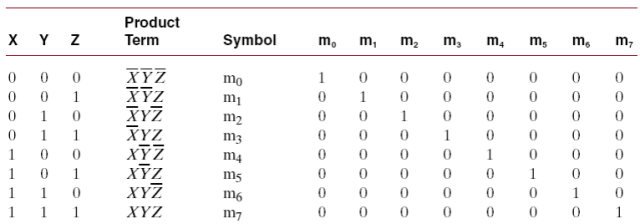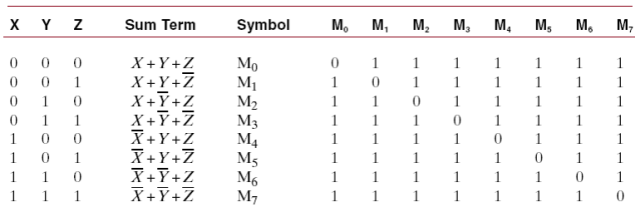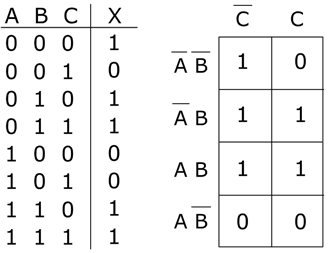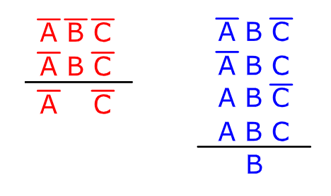## Register Now

Lorem ipsum dolor sit amet, consectetur adipiscing elit.Morbi adipiscing gravdio, sit amet suscipit risus ultrices eu.Fusce viverra neque at purus laoreet consequa.Vivamus vulputate posuere nisl quis consequat.

K-Map or Karnaugh Map is a graphical representation of input conditions similar to truth table. It is an array of cells which helps in logical simplification or minimization of Boolean expression. This post will discuss about Karnaugh Map (K-Map), simplification of Boolean expression, Minterm, Maxterm, its applications, advantages and disadvantages.

## What is K-Map (Karnaugh Map)

Karnaugh Map is a two-dimensional graphical representation of the input and output conditions which can minimize the Boolean expression involving 2, 3, 4 and 5 variables. In other words, it is used to remove redundant operations in a Boolean function. It is named after Maurice Karnaugh, an American mathematician and physicist, who developed K- Map at Harvard Computation Laboratory.Fig. 1 – Maurice Karnaugh, Founder of Karnaugh Map

Fig. 2 below shows an example of Karnaugh Map with two variables and each square is called a ‘cell’. Four cells are numbered (0, 1, 2, 3). K-Map having n-variable has 2n cells where in each cell corresponds to the truth table value. The cells are designated with a value that corresponds to truth-table row. The cells are arranged such that the adjacent cells differ by only one bit position.Fig. 2 – Example of Karnaugh Map (K-Map)

## What is Minterm and Maxterm

Let us understand the meaning of Minterm and Maxterm in this section as it helps in the simplification of Boolean expression using K- Map.

### Minterm

Minterm of ‘n’ variables is a product of ‘n’ variables which appear exactly once in True or Complemented form.

Example: Minterms of 3 variables(X,Y,Z) = Each Minterm = 1 for only one combination of values of the variables it represents otherwise 0. Identifying the Minterms from the K-map is equivalent to reading equations in Sum-of-Minterms or Sum-of-Products (SOP) form, directly from the truth table.Fig. 3 – Minterms for Three Variables

### Maxterm

Maxterm of ‘n’ variables is a sum of ‘n’ variables which appear exactly once in True or Complemented form.

Example: Maxterm of 3 variables (X,Y,Z)= Each Maxterm = 0 for only one combination of values of the variables it represents otherwise the value is 1. Hence Boolean function could be expressed algebraically from a given truth table by identifying the logical product of all the Maxterms that produce 0 in the function. This expression is called as Product of Maxterms or Product-of-Sums (POS)Fig. 4 – Maxterms for Three Variables

## Simplification of Boolean Expression Using K-Map (Karnaugh Map)

Simplification of Boolean expression using K- Map requires grouping of 1’s. Grouping of 1’s should follow certain rules:

• Groups should have 2, 4, 8 one’s (1’s).
• Identification of Minterms or Maxterms is necessary.
• Grouping is done horizontally, vertically but never diagonally.
• Multiple groups using repeated 1’s can be done.

This can be better explained with an example using 3 variables as shown below:Two groups are formed: Group 1 is marked in Blue Color and the other group in Red Color. The groups are listed below.The variables that are remaining are OR’ed (Logical Addition) to obtain the reduced Boolean expression.## Applications of Karnaugh Map (K-Map)

The applications include:

• They are used in design and implementation of Digital Circuits
• K- Maps are used in the simplification of Half- Adder and Full- Adder circuits

• The most economical and simplified circuit can be built using K-Maps.
• Using K-Maps, logical operation is fast.
• Deriving at the simplest expression helps in reduction of instructions in software applications.
• It is more efficient than any other simplification techniques of Boolean algebra.

```Also Read: